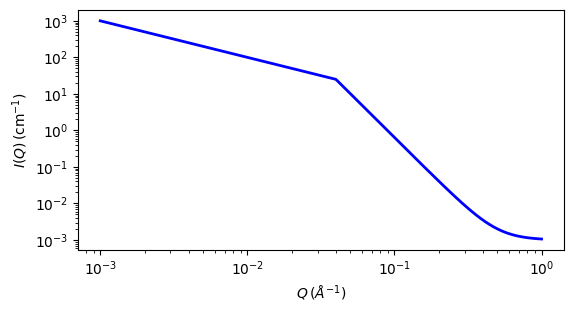# two_power_law

This model calculates an empirical functional form for SAS data characterized by two power laws.

Parameter

Description

Units

Default value

scale

Scale factor or Volume fraction

None

1

background

Source background

cm-1

0.001

coefficent_1

coefficent A in low Q region

None

1

crossover

crossover location

-1

0.04

power_1

power law exponent at low Q

None

1

power_2

power law exponent at high Q

None

4

The returned value is scaled to units of cm-1 sr-1, absolute scale.

Definition

The scattering intensity $$I(q)$$ is calculated as

$\begin{split}I(q) = \begin{cases} A q^{-m1} + \text{background} & q <= q_c \\ C q^{-m2} + \text{background} & q > q_c \end{cases}\end{split}$

where $$q_c$$ = the location of the crossover from one slope to the other, $$A$$ = the scaling coefficient that sets the overall intensity of the lower Q power law region, $$m1$$ = power law exponent at low Q, and $$m2$$ = power law exponent at high Q. The scaling of the second power law region (coefficient C) is then automatically scaled to match the first by following formula:

$C = \frac{A q_c^{m2}}{q_c^{m1}}$

Note

Be sure to enter the power law exponents as positive values!

For 2D data the scattering intensity is calculated in the same way as 1D, where the $$q$$ vector is defined as

$q = \sqrt{q_x^2 + q_y^2}$Fig. 124 1D plot corresponding to the default parameters of the model.

Source

two_power_law.py

References

None.

Authorship and Verification

• Author: NIST IGOR/DANSE Date: pre 2010